## 李荣浩求婚成功你酸了吗？全新哈弗H6智能贴心陪伴

2019年07月11日 20:18 来源：车行天下 超过：14336次关注

身边总有人讨论：单身好还是恋爱好?

对于这个问题，我曾倔强地认为自己没有标准答案。在我看来，恋爱是两个人的欢喜，单身意味着自主自由，两者春兰秋菊，各有所长，但直到最近我才发现其实不然。

近日一位朋友在考虑换辆新车，几经对比考察，终于找到了自己的心仪之选，并推荐給也有换车念头的我。于是在她的强烈安利下，我认识并入手了它——全新哈弗H6。有了这个“小伙伴”相陪，才发现，原来以前的归途是如此冷清，原来曾经独身的我是如此孤独，原来，一个人的路途也可以如此欢乐。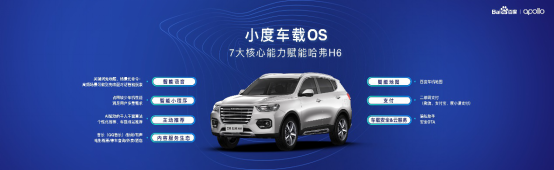曾经，我总想着，路途上随便说说话，哪怕能有句淡然的回复“我在”，也定能让我感到温馨，可全新哈弗H6给我的却不仅仅是一句“我在”。由于搭载了采用百度语音识别方案，结合富迪FM1388硬降噪、拥有70+语义垂类的全新智能网联系统，它能懂我所想，知我所要。感到口渴，说一句“你好哈弗，我渴了”，就会马上推荐附近的饮品店;感到疲惫，说一句“好累呀”，就会主动关怀是否需要到最近旅店或停车场进行休息;感到炎热，说一句“我有点热”，空调便会马上开启。此外，它还可以汇报天气、陪我闲聊、给我讲笑话、和我玩成语接龙……全新哈弗H6给了我全方位的陪伴。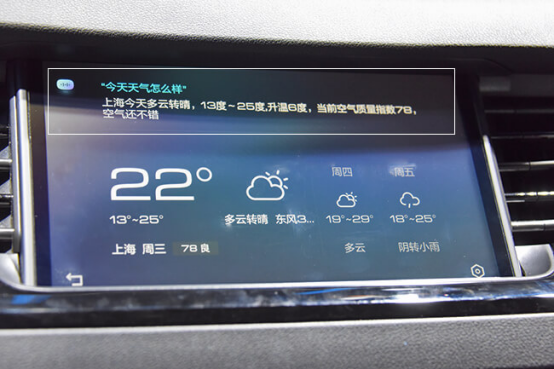曾经，我无比担心一个人开车去往远方，每次出门，路的方向总让我迷茫，有时甚至能绕个好几圈。然而入手了全新哈弗H6后，我仿佛就拥有了一个超强的“引路人”。基于百度地图在国内超过5000w的海量poi数据资源，全新哈弗H6车机系统内的3D楼块更直观，沉浸感更强且位置十分精确，给了我一个人追寻“诗和远方”的勇气和现实条件。无论是信号弱的隧道还是地下停车场，“引路人”依旧能陪伴在我身边，给我正常的定位数据。我们之间的交流用语音也能实现，即使是超长问题“我要从四惠出发去北京西站然后在去西单大悦城，帮我规划一条红绿灯少不堵车的最快路线”，它也能秒懂，并给我最优路线，可以说，我们之间的沟通是无障碍的，有了它我终于也不再是那个迷糊的路痴了!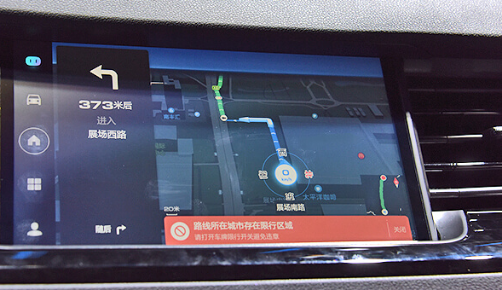曾经，我认为一个人的路途必定是缺少娱乐性的，时下流行的剧目、歌曲等资源很难在行车中出现，乏味而又孤单。可全新哈弗H6却改变了我的刻板印象，无论最新一期《乐队的夏天》中的单曲、迪士尼电影《花木兰》主题曲还是吴青峰的全新单曲《巴别塔庆典》，都可随心而听;无论是《长安十二时辰》的原著小说、民间野史奇闻怪谈还是搞笑相声，都可任意倾听;无论是开播的《亲爱的，热爱的》电视剧、综艺《向往的生活》第三季还是仍在连载的动漫《名侦探柯南》，也都可以随意观看。能够全部实现以上内容，只因它的全新智能网联系统拥有2000万+在线版权音乐、海量喜马拉雅资源以及百度视频、爱奇艺、搞笑视频等多个在线视频平台。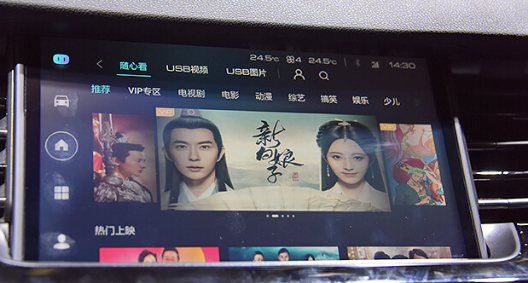曾经，因为极度缺乏安全感但很多时候又只能独自一人而让我一度畏惧开车出游。可搭载全新智能网联系统的全新哈弗H6却给了我勇气，让我产生信任感。它拥有I-Call (专属人工坐席)、B-Call (一键道路救援)、E-Call (紧急救援)、白金10分钟自救互救指导及MPDS等十多项比较完整的智能救援服务，旅途中无论出现什么情况，都可以在第一时间获得救助，让我安心不少。当车辆车辆离开设定范围，智能监控系统就能通过为车辆设定电子围栏的手机APP给我发出警报，即使车门异常打开时也能收到提醒短信，犹如男朋友一般时刻贴心守护。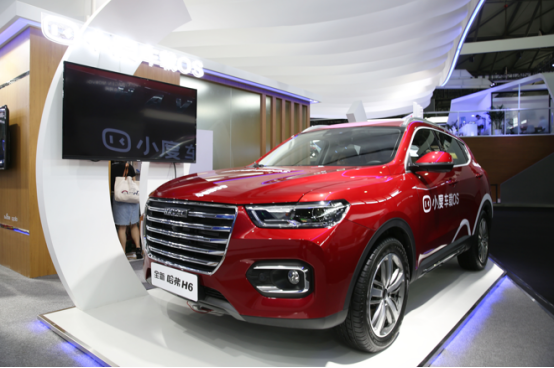曾经，我没想过车辆智能系统里还能有如百度贴吧、携程旅行、小红书等这样的小程序，而全新哈弗H6里各类场景应用几乎都有，它用它那强大的智能系统陪伴着我出行，帮我克服孤独。不仅如此，它还打破了我对于热销商品的质疑。哈弗H6在SUV中的销量表现十分抢眼，6月份以27052辆的优异成绩上榜，且已经是累计73个月第一了。在全新哈弗H6的陪伴里，我清醒地认识到，销量高与其实力着实是密切相关的。曾经我也没想到，本就已经具有极高性价的全新哈弗H6竟还能有这样的优惠——“真国六 放心购 哈弗钜惠至高2.8万”的促销活动，活动期间，全新哈弗H6至高可享综合钜惠1.8万元;“哈弗邀您团战一夏”活动中还能以低价享受车辆养护、空调养护等项目，论坛发帖还有机会获得积分，可用来兑换工时券。原来，想要一个贴心陪伴的智能“小伙伴”也没有那么难嘛!

#### 相关文章

0-500 字已有评论 0条 查看评论>>

### 热门标签

﻿
• 快速找车
• 选择品牌
• 选择品牌
• A  奥迪
• A  阿斯顿·马丁
• A  阿尔法·罗密欧
• B  宝沃
• B  布加迪
• B  巴博斯
• B  保时捷
• B  宾利
• B  奔驰
• B  宝马
• B  本田
• B  别克
• B  标致
• B  比亚迪
• B  宝骏
• B  北汽制造
• B  北汽新能源
• B  北汽幻速
• B  北汽威旺
• B  北京汽车
• B  奔腾
• B  北汽绅宝
• C  长安
• C  长安商用
• C  长城
• C  昌河
• D  大众
• D  道奇
• D  DS
• D  东南
• D  东风风神
• D  东风风行
• D  东风小康
• D  东风风度
• D  东风
• F  福特
• F  丰田
• F  菲亚特
• F  法拉利
• F  福田
• F  福迪
• F  福汽启腾
• G  观致
• G  广汽传祺
• G  广汽吉奥
• G  GMC
• H  红旗
• H  汉腾汽车
• H  哈弗
• H  哈飞
• H  海格
• H  海马
• H  华颂
• H  黄海
• H  华泰
• H  恒天
• J  吉利汽车
• J  捷豹
• J  Jeep
• J  江淮
• J  江铃
• J  金杯
• J  九龙
• J  金旅
• K  凯翼
• K  凯迪拉克
• K  克莱斯勒
• K  科尼塞克
• K  卡威
• K  开瑞
• L  路虎
• L  林肯
• L  劳斯莱斯
• L  兰博基尼
• L  雷克萨斯
• L  铃木
• L  雷诺
• L  理念
• L  力帆
• L  莲花汽车
• L  猎豹
• L  路特斯
• L  陆风
• M  马自达
• M  MG
• M  MINI
• M  玛莎拉蒂
• M  摩根
• M  迈凯轮
• N  纳智捷
• O  欧宝
• O  讴歌
• O  欧朗
• Q  奇瑞
• Q  起亚
• Q  启辰
• R  日产
• R  荣威
• R  瑞麒
• S  三菱
• S  斯威汽车
• S  萨博
• S  smart
• S  斯柯达
• S  斯巴鲁
• S  思铭
• S  双龙
• S  上汽大通
• S  双环
• T  特斯拉
• T  腾势
• W  沃尔沃
• W  五菱汽车
• W  五十铃
• W  威兹曼
• W  威麟
• X  现代
• X  雪佛兰
• X  雪铁龙
• X  西雅特
• Y  一汽
• Y  英菲尼迪
• Y  英致
• Y  依维柯
• Y  野马汽车
• Y  永源
• Z  众泰
• Z  中华
• Z  中兴
• Z  知豆
• 选择车系
• 选择车系
• 车型对比
• 选择品牌
• 选择品牌
• A  奥迪
• A  阿斯顿·马丁
• A  阿尔法·罗密欧
• B  宝沃
• B  布加迪
• B  巴博斯
• B  保时捷
• B  宾利
• B  奔驰
• B  宝马
• B  本田
• B  别克
• B  标致
• B  比亚迪
• B  宝骏
• B  北汽制造
• B  北汽新能源
• B  北汽幻速
• B  北汽威旺
• B  北京汽车
• B  奔腾
• B  北汽绅宝
• C  长安
• C  长安商用
• C  长城
• C  昌河
• D  大众
• D  道奇
• D  DS
• D  东南
• D  东风风神
• D  东风风行
• D  东风小康
• D  东风风度
• D  东风
• F  福特
• F  丰田
• F  菲亚特
• F  法拉利
• F  福田
• F  福迪
• F  福汽启腾
• G  观致
• G  广汽传祺
• G  广汽吉奥
• G  GMC
• H  红旗
• H  汉腾汽车
• H  哈弗
• H  哈飞
• H  海格
• H  海马
• H  华颂
• H  黄海
• H  华泰
• H  恒天
• J  吉利汽车
• J  捷豹
• J  Jeep
• J  江淮
• J  江铃
• J  金杯
• J  九龙
• J  金旅
• K  凯翼
• K  凯迪拉克
• K  克莱斯勒
• K  科尼塞克
• K  卡威
• K  开瑞
• L  路虎
• L  林肯
• L  劳斯莱斯
• L  兰博基尼
• L  雷克萨斯
• L  铃木
• L  雷诺
• L  理念
• L  力帆
• L  莲花汽车
• L  猎豹
• L  路特斯
• L  陆风
• M  马自达
• M  MG
• M  MINI
• M  玛莎拉蒂
• M  摩根
• M  迈凯轮
• N  纳智捷
• O  欧宝
• O  讴歌
• O  欧朗
• Q  奇瑞
• Q  起亚
• Q  启辰
• R  日产
• R  荣威
• R  瑞麒
• S  三菱
• S  斯威汽车
• S  萨博
• S  smart
• S  斯柯达
• S  斯巴鲁
• S  思铭
• S  双龙
• S  上汽大通
• S  双环
• T  特斯拉
• T  腾势
• W  沃尔沃
• W  五菱汽车
• W  五十铃
• W  威兹曼
• W  威麟
• X  现代
• X  雪佛兰
• X  雪铁龙
• X  西雅特
• Y  一汽
• Y  英菲尼迪
• Y  英致
• Y  依维柯
• Y  野马汽车
• Y  永源
• Z  众泰
• Z  中华
• Z  中兴
• Z  知豆
• 选择车系
• 选择车系
• 选择车型
• 选择车型
• 意见反馈